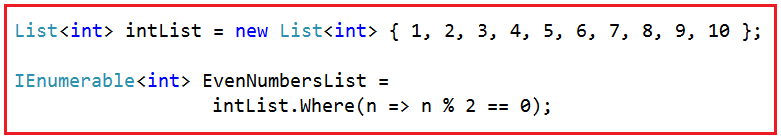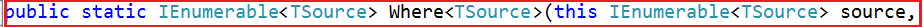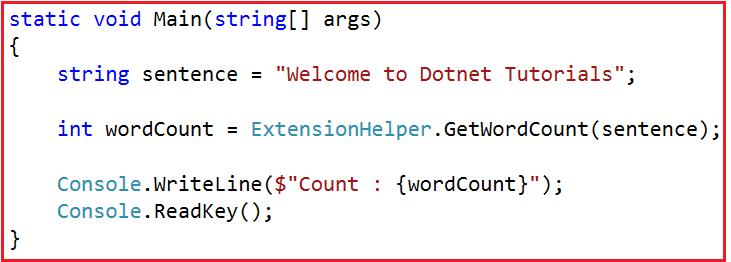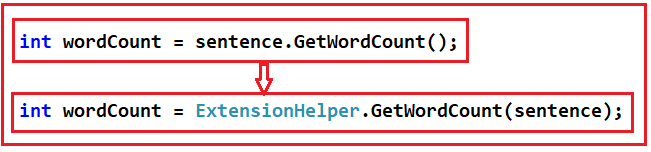# LINQ Extension Methods in C#

## LINQ Extension Methods in C# with Examples

In this article, I will discuss the LINQ Extension Methods in C# with Examples. Please read our previous article before proceeding to this article, where we discussed the Differences between IEnumerable and IQueryable in C#. At the end of this article, you will understand the following three concepts in C#.

1. What are Extension Methods in C#?
2. When to use Extension Methods in C#?
3. How to Implement Extension Methods in C#?
4. Understanding the LINQ Extension Methods.
##### What are LINQ Extension Methods?

The LINQ’s standard query operators, such as select, where, etc., are implemented in the Enumerable class. These methods are implemented as extension methods of the type IEnumerable<T> interface. Let us understand this with an example. We have the following code in our Main method.The above Where() method does not belong to the List<T> class, but still, we can call it as it belongs to the List<T> class. Why can it be called using the List<T> object? Let’s find out. If you go to the definition of the Where method, then you will find the following definition.As you can see in the signature, the where Where() method is implemented as an extension method on the IEnumerable<T> interface, and we know List<T> implements the IEnumerable<T> interface. This is the reason why we can call the Where() method using the List<T> object. With this in mind, let us understand what extension methods are and how they are implemented in C#.

##### What are Extension Methods in C#?

According to MSDN, Extension methods allow us to add methods to existing types without creating a new derived type, recompiling, or modifying the original type.

In simple words, we can say that the Extension methods can be used as an approach to extending the functionality of a class by adding new methods into the existing class if the source code of the class is not available or if we don’t have any permission in making changes to the existing class.

The most important point you need to remember is that extension methods are the static methods of a static class, but they will be called as if they were instance methods on the extended type.

##### When to Use Extension Methods in C#?

You need to use an extension method if any of the following conditions are true:

1. You need a method on an existing type, and you are not the owner of the source code of that type.
2. You need a method on an existing type; you do not own the source code of that type, but that type is an interface.
3. You need a method on an existing type, you do not own the source code, and that type is not an interface, but adding the method creates undesired coupling.

Otherwise, you should go with the normal method of the actual type itself.

##### How to Implement Extension Methods in C#?

Let us understand this with an example. Our requirement is that we want to add a method in the built-in string class. Let’s call this method GetWordCount(), which will count the word present in a string separated by a space.

For example, if the string is “Welcome to Dotnet Tutorials,” it should return the word count as 4. The most important point is that we need to call this method on the String object, as shown below.
int wordCount = sentence.GetWordCount();

Note: We cannot define the GetWordCount() method directly in the string class as we do not own the string class. The string class belongs to the System namespace, owned by the .NET framework. So, the alternative solution to achieve this is to write a wrapper class, as shown below.

```public class ExtensionHelper
{
public static int GetWordCount(string str)
{
if (!String.IsNullOrEmpty(str))
return str.Split(' ').Length;
return 0;
}
}
```

The above ExtensionHelper Wrapper class works fine, but the problem is that we cannot call the GetWordCount() method using the string object, as shown below.
int wordCount = sentence.GetWordCount();

Instead, we need to call the GetWordCount() method, as shown below.
int wordCount = ExtensionHelper.GetWordCount(sentence);

##### How to convert the above GetWordCount() method to an Extension Method of the String class?

Now, let’s convert the GetWordCount() method to an extension method on the String class. So that we can able to call the GetWordCount() method using the following syntax.
int wordCount = sentence.GetWordCount();

To make the above GetWordCount() method an extension method, we need to make the following changes.

1. First, we need to make the ExtensionHelper class a static class.
2. Second, the type the method extends (i.e., string) should be passed as the first parameter preceding the “this” keyword to the GetWordCount() method.

With the above two changes in place, the GetWordCount() method becomes an extension method, and hence, we can call the GetWordCount() method in the same way as we call an instance method of a class. The complete example code is given below.

```using System;
namespace LINQDemo
{
class Program
{
static void Main(string[] args)
{
string sentence = "Welcome to Dotnet Tutorials";

int wordCount = sentence.GetWordCount();

Console.WriteLine(\$"Count : {wordCount}");
}
}

public static class ExtensionHelper
{
public static int GetWordCount(this string str)
{
if (!String.IsNullOrEmpty(str))
return str.Split(' ').Length;
return 0;
}
}
}
```

Now run the application, and you will see the word count as expected in the console window. Here, we can still call the GetWordCount() extension method using the wrapper class style syntax and get the output as expected, as shown below.So, the point that I need to keep focus on is that this is how the extension methods are called internally behind the scenes.That means it is also possible to call the LINQ extension methods such as select, where, etc., using the wrapper class style syntax. As all the LINQ extension methods are implemented in the Enumerable class, the syntax to call those methods should look as shown below.

```using System;
using System.Collections.Generic;
using System.Linq;

namespace LINQDemo
{
class Program
{
static void Main(string[] args)
{
List<int> intList = new List<int> { 1, 2, 3, 4, 5, 6, 7, 8, 9, 10 };
IEnumerable<int> EvenNumbers = Enumerable.Where(intList, n => n % 2 == 0);

}
}
}
```
##### LINQ Extension Method in C#:

LINQ (Language Integrated Query) extension methods in C# are a set of methods provided by the System.Linq namespace that extends the capabilities of collections (like arrays, lists, and other types implementing IEnumerable<T>) by adding query functionality. These methods enable querying collections using a SQL-like syntax directly in C#. Here’s an overview of some commonly used LINQ extension methods:

Where: Filters a sequence based on a predicate.
var filteredResult = collection.Where(item => item.Property == someValue);

Select: Projects each element of a sequence into a new form.
var projectedResult = collection.Select(item => new { item.Property1, item.Property2 });

OrderBy / OrderByDescending: Sorts the elements of a sequence in ascending or descending order.
var sortedResult = collection.OrderBy(item => item.Property);
var sortedDescendingResult = collection.OrderByDescending(item => item.Property);

GroupBy: Groups the elements of a sequence.
var groupedResult = collection.GroupBy(item => item.Property);

FirstOrDefault / LastOrDefault: Returns the first or last element of a sequence or a default value if no element is found.
var firstItem = collection.FirstOrDefault();
var lastItem = collection.LastOrDefault();

Sum / Min / Max / Average: Computes the sum, minimum, maximum, or average of a sequence.
var total = collection.Sum(item => item.NumericProperty);

Any / All: Checks if any or all sequence elements satisfy a condition.
bool hasItems = collection.Any();
bool allMatchCondition = collection.All(item => item.Property > someValue);

Distinct: Returns distinct elements from a sequence.
var distinctItems = collection.Distinct();

Count: Counts the elements in a sequence, optionally matching a specific condition.
int count = collection.Count();
int conditionalCount = collection.Count(item => item.Property == someValue);

Intersect / Except / Union: Performs set operations like intersection, difference, and union on sequences.
var commonElements = collection1.Intersect(collection2);

In the next article, I will discuss the LINQ Operators in C# with Examples. In this article, I try to explain the Linq Extension Methods in C# with Examples. I hope you enjoy this LINQ Extension Methods in C# article.